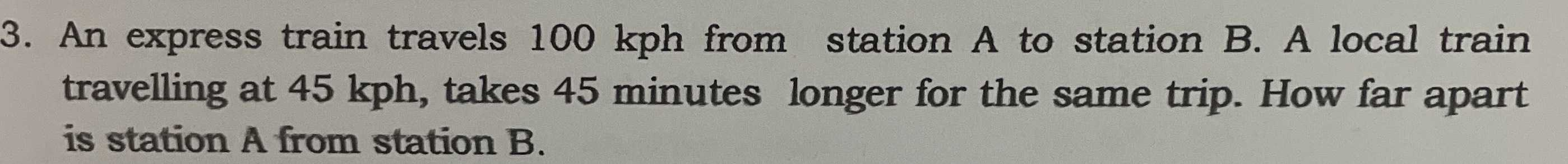### ¿Todavía tienes preguntas de matemáticas?

Pregunte a nuestros tutores expertos
Algebra
Pregunta3. An express train travels $$100 kph$$ from station A to station B. A local train travelling at $$45 kph$$ , takes $$45$$ minutes longer for the same trip. How far apart is station A from station B.

Let "d" be this distance.

The "time equation" is-=of an hour  (= 45 minutes).

To solve it, multiply both sides by 900.

You will get    20d - 9d = 675

11d    = 675

d    == 61.364.

CHECK.= 0.75 =of an hour

Solución
View full explanation on CameraMath App.